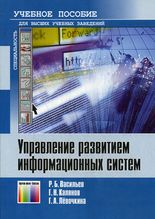• A
• A
• A
• ABC
• ABC
• ABC
• А
• А
• А
• А
• А
Regular version of the site
Of all publications in the section: 8
Sort:
by name
by year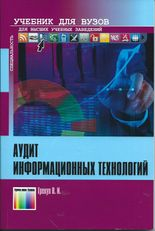Book
Грекул В. И. М.: Научно-техническое издательство «Горячая линия – Телеком», 2015.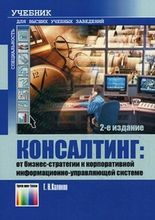Book
Калянов Г. Н. М.: Научно-техническое издательство «Горячая линия – Телеком», 2011.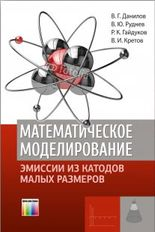Book
Данилов В. Г., Руднев В. Ю., Гайдуков Р. К. и др. М.: Научно-техническое издательство «Горячая линия – Телеком», 2014.

A new mathematical model of heat transfer in silicon field emission pointed cathode of small dimensions is constructed which permits taking its partial melting into account. This mathematical model is based on the phase field system, i.e., on a contemporary generalization of Stefan-type problems. The approach used by the authors is not purely mathematical but is based on the understanding of the solution structure (construction and study of asymptotic solutions) and computer calculations. The book presents an algorithm for numerical solution of the equations of the obtained mathematical model including its parallel implementation. The results of numerical simulation conclude the book.

The book is intended for specialists in the field of heat transfer and field emission processes and can be useful for senior students and postgraduates.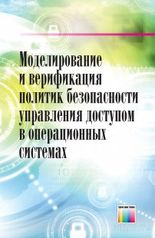Book
Девянин П. Н., Ефремов Д. В., Кулямин В. В. и др. М.: Научно-техническое издательство «Горячая линия – Телеком», 2019.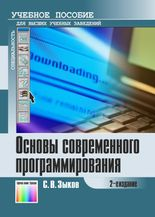Book
Зыков С. В. М.: Научно-техническое издательство «Горячая линия – Телеком», 2012.